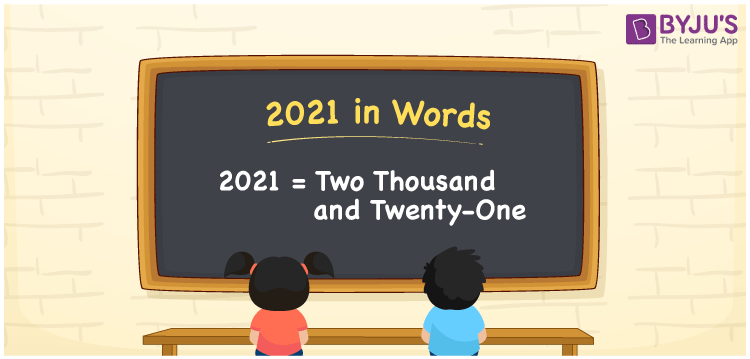# 2021 in Words

We can write 2021 in words as Two thousand and twenty-one. We can effortlessly emanate the word form of 2021 with the help of a place value chart. The word form of 2021 is also called the number name of 2021. For example, if you see the number 2021 on a screen, you may spell it as “Two thousand and twenty-one” or “Two thousand twenty-one”. In the same way, we can write any given cardinal number in words.

 2021 in words Two thousand and twenty-one Two thousand and twenty-one in Numbers 2021

## 2021 in English Words

We generally express numbers in words using the English alphabet. Thus, we can spell 2021 in English as “Two thousand and twenty-one”.## How to Write 2021 in Words?

Let’s learn how to derive the number 2021 in words using a four-column place value chart since it is a four-digit number. The below table represents the place values for all the four digits of the given number, i.e., 2021.

 Thousands Hundreds Tens Ones 2 0 2 1

Here, ones = 1, tens = 2, hundreds = 0, and thousands = 2.

Thus, we can write the expanded form as:

2 × Thousand + 0 × Hundred + 2 × Ten + 1 × One

= 2 × 1000 + 0 × 100 + 2 × 10 + 1 × 1

= 2000 + 20 + 1

= Two thousand + Twenty-one {since twenty + one = twenty-one}

= Two thousand and twenty-one

Therefore, 2021 in words is written as Two thousand and twenty-one.

2021 is a natural number that precedes 2022 and succeeds 2020.

2021 in words – Two thousand and twenty-one

Is 2021 an even number? – No

Is 2021 an odd number? – Yes

Is 2021 a prime number? – No

Is 2021 a composite number? – Yes

Is 2021 a perfect square number? – No

Is 2021 a perfect cube number? – No

## Frequently Asked Questions on 2021 in Words

Q1

### How do you write the year 2021 in words?

We can write the year 2021 in words as “The year two thousand and twenty-one”.
Q2

### How to write Rs. 2021 in words on a cheque?

On a cheque, we can write Rs. 2021 in words as “Two thousand and twenty-one rupees only”.
Q3

### What is the value of 1800 + 221 in words?

1800 + 221 = 2021 Therefore, the value of 1800 + 221, i.e. 2021 in words is Two thousand and twenty-one.